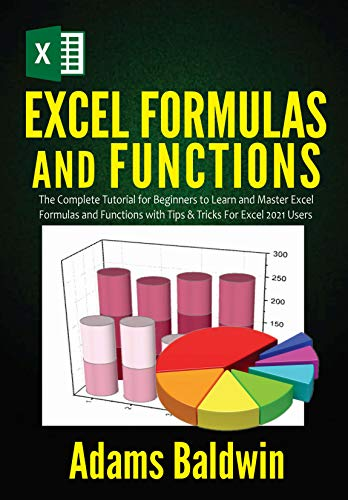Excel Formulas and Functions: The Complete Tutorial for Beginners to Learn and Master Excel Formulas and Functions with Tips & Tricks For Excel 2021 Users - THE PIRATE BOOK

## Tuesday, June 22, 2021# Excel Formulas and Functions: The Complete Tutorial for Beginners to Learn and Master Excel Formulas and Functions with Tips & Tricks For Excel 2021 Users

• Length: 81 pages
• Edition: 1
• Publication Date: 2021-03-11
• ISBN-10: B08YRQL5LX
Description

This book on Excel Formulas and Functions for Beginners 2021 is a perfect guide for complete beginners or casual spreadsheet users. With patience and by following the simple step-by-step process, you will learn how to work with Excel at the office or at home for personal reasons.
The purpose of this beginner’s guide is to explain to you how and when to use all the powerful tools and features Excel offers.
You shouldn’t be stressing yourself to memorize functions and formulas because Excel can do that heavy lifting for you.

Here are the things you will get the chance of learning in this book:

• Benefits of using Excel
• Functions and Formulas in Excel
• How to Enter a Formula?
• How to Edit a Formula?
• Precedence of Operators
• How to Copy or Paste a Formula?
• How to Insert a Function?
• The Formula Tab
• Relative References
• How to Create and Copy the Formula Using a Relative Reference
• Absolute References
• How to Create and Copy Formula Using Absolute Referencing?
• Mixed Cell Referencing
• Future Value
• FVSCHEDULE
• Present Value
• Net PresentValue
• Using the AND function
• BETWEEN Condition
• IF Function
• IFS Function
• IFERROR Function
• OR Function
• Date Function
• More Functions
• Statistica lFunctions
• Computing GDPs
• Currency
• Decimal Places
• Editing Text with Formulas
• Number of Characters
• Counting Numbers
• IF Statements
• Looking for Information
• Time and Date
• AutoFill
• Text to Columns
• Transpose
• Automatically Sum Everything
• Work on Different Sheets
• Display Formulas
• Excel Tips and Tricks
• And many more….
#evba #kingexcel #etipfree #eama